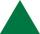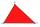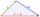3-bracket 2

May be the smallest angle in the triangle greater than 40°?

Result

Solution:Leave us a comment of example and its solution (i.e. if it is still somewhat unclear...):Be the first to comment!Next similar examples:

1. 3-bracketMay be the largest angle in the triangle less than 20°?
2. 3-bracket 3Two angles in a triangle are 90° and 60°. Has triangle at least two equal sides?
3. Angles 1It is true neighboring angles have not common arm?
4. Triangle P2Can triangle have two right angles?
5. QuizQAn isosceles triangle has two sides of length 7 km and 39 km. How long is a third side?
6. TriangleFor how many integer values of x can 16, 15 and x be the lengths of the sides of triangle?
7. MedianThe median of the triangle LMN is away from vertex N 84 cm. Calculate the length of the median, which start at N.
8. TriangleDetermine if it is possible to construct a triangle with sides 28 31 34 by calculation.
9. Center traverseIt is true that the middle traverse bisects the triangle?
10. TriangleProve whether you can construct a triangle ABC, if a=9 cm, b=10 cm, c=4 cm.
11. ExpressionSolve for a specified variable: P=a+4b+3c, for a
12. SimplifySimplify the following problem and express as a decimal: 5.68-[5-(2.69+5.65-3.89) /0.5]
13. Bus 14Boatesville is 65.35 kilometers from Stanton. A bus traveling from Stanton is 24.13 kilometers from Boatesville. How far has the bus traveled?
14. Fraction to decimalWrite the fraction 3/22 as a decimal.
15. ZdeněkZdeněk picked up 15 l of water from a 100-liter full-water barrel. Write a fraction of what part of Zdeněk's water he picked.
16. Lengths of the poolMiguel swam 6 lengths of the pool. Mat swam 3 times as far as Miguel. Lionel swam 1/3 as far as Miguel. How many lengths did mat swim?
17. Mixed2improperWrite the mixed number as an improper fraction. 166 2/3